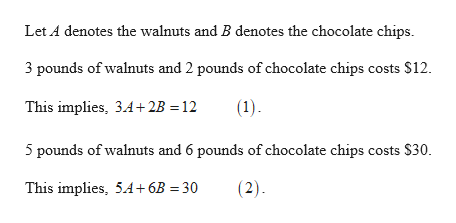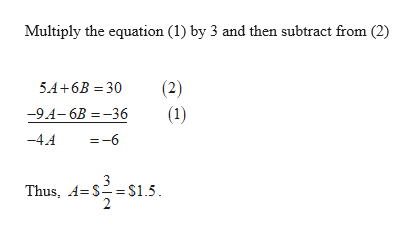# A store is having a sale on walnuts and chocolate chips. For3pounds of walnuts and2pounds of chocolate chips, the total cost is\$12. For5pounds of walnuts and6pounds of chocolate chips, the total cost is\$30. Find the cost for each pound of walnuts and each pound of chocolate chips.

Question
14 views

A store is having a sale on walnuts and chocolate chips. For

3

pounds of walnuts and

2

pounds of chocolate chips, the total cost is

\$12

. For

5

pounds of walnuts and

6

pounds of chocolate chips, the total cost is

\$30

. Find the cost for each pound of walnuts and each pound of chocolate chips.

check_circle

Step 1help_outlineImage TranscriptioncloseLet A denotes the walnuts and B denotes the chocolate chips. 3 pounds of walnuts and 2 pounds of chocolate chips costs \$12. This implies, 3.4+2B =12 (1). 5 pounds of walnuts and 6 pounds of chocolate chips costs \$30. This implies, 5.A+6B = 30 (2). fullscreen
Step 2help_outlineImage TranscriptioncloseMultiply the equation (1) by 3 and then subtract from (2) (2) (1) 5A+6B = 30 -94-6B = -36 -4.4 =-6 Thus, 4= \$== \$1.5. fullscreen

### Want to see the full answer?

See Solution

#### Want to see this answer and more?

Solutions are written by subject experts who are available 24/7. Questions are typically answered within 1 hour.*

See Solution
*Response times may vary by subject and question.
Tagged in

### Equations and In-equations